## Complement Of Graph-

Complement of a simple graph G is a simple graph G’ having-

• All the vertices of G.
• An edge between two vertices v and w iff there exists no edge between v and w in the original graph G.

## Complement Of Graph Example-

The following example shows a graph G and its complement graph G’-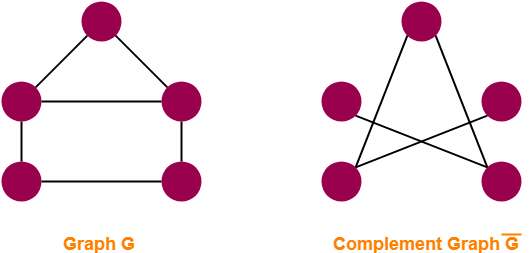## Relationship Between G & G’-

The following relationship exists between a graph G and its complement graph G’-

1. Number of vertices in G = Number of vertices in G’.

 |V(G)| = |V(G’)|

2. The sum of total number of edges in G and G’ is equal to the total number of edges in a complete graph.

 |E(G)| + |E(G’)|= C(n,2)= n(n-1) / 2

where n = total number of vertices in the graph

## Important Terms-

It is important to note the following terms-

• Order of graph = Total number of vertices in the graph
• Size of graph = Total number of edges in the graph

Also Read-Types of Graphs in Graph Theory

## Problem-01:

A simple graph G has 10 vertices and 21 edges. Find total number of edges in its complement graph G’.

## Solution-

Given-

• Number of edges in graph G, |E(G)| = 21
• Number of vertices in graph G, n = 10

We know |E(G)| + |E(G’)| = n(n-1) / 2.

Substituting the values, we get-

21 + |E(G’)| = 10 x (10-1) / 2

|E(G’)| = 45 – 21

∴ |E(G’)| = 24

Thus, Number of edges in complement graph G’ = 24.

## Problem-02:

A simple graph G has 30 edges and its complement graph G’ has 36 edges. Find number of vertices in G.

## Solution-

Given-

• Number of edges in graph G, |E(G)| = 30
• Number of edges in graph G’, |E(G’)| = 36

We know |E(G)| + |E(G’)| = n(n-1) / 2.

Substituting the values, we get-

30 + 36 = n(n-1) / 2

n(n-1) = 132

n2 – n – 132 = 0

Solving this quadratic equation, we get n = 12.

Thus, Number of vertices in graph G = 12.

## Problem-03:

Let G be a simple graph of order n. If the size of G is 56 and the size of G’ is 80. What is n?

## Solution-

Size of a graph = Number of edges in the graph.

Given-

• Number of edges in graph G, |E(G)| = 56
• Number of edges in graph G’, |E(G’)| = 80

We know |E(G)| + |E(G’)| = n(n-1) / 2.

Substituting the values, we get-

56 + 80 = n(n-1) / 2

n(n-1) = 272

n2 – n – 272 = 0

Solving this quadratic equation, we get n = 17.

Thus, Number of vertices in graph G = 17.

In other words, Order of graph G = 17.

To gain better understanding about Complement Of Graph,

Watch this Video Lecture

Next Article-Walks in Graph Theory

Get more notes and other study material of Graph Theory.

Watch video lectures by visiting our YouTube channel LearnVidFun.

## Graph Isomorphism-

 Graph Isomorphism is a phenomenon of existing the same graph in more than one forms.Such graphs are called as Isomorphic graphs.

### Graph Isomorphism Example-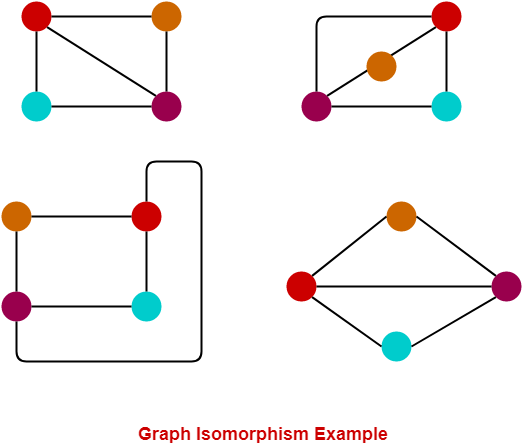Here,

• The same graph exists in multiple forms.
• Therefore, they are Isomorphic graphs.

## Graph Isomorphism Conditions-

For any two graphs to be isomorphic, following 4 conditions must be satisfied-

• Number of vertices in both the graphs must be same.
• Number of edges in both the graphs must be same.
• Degree sequence of both the graphs must be same.
• If a cycle of length k is formed by the vertices { v1 , v2 , ….. , vk } in one graph, then a cycle of same length k must be formed by the vertices { f(v1) , f(v2) , ….. , f(vk) } in the other graph as well.

### Degree Sequence

Degree sequence of a graph is defined as a sequence of the degree of all the vertices in ascending order.

## Important Points-

• The above 4 conditions are just the necessary conditions for any two graphs to be isomorphic.
• They are not at all sufficient to prove that the two graphs are isomorphic.
• If all the 4 conditions satisfy, even then it can’t be said that the graphs are surely isomorphic.
• However, if any condition violates, then it can be said that the graphs are surely not isomorphic.

## Sufficient Conditions-

The following conditions are the sufficient conditions to prove any two graphs isomorphic.

If any one of these conditions satisfy, then it can be said that the graphs are surely isomorphic.

• Two graphs are isomorphic if and only if their complement graphs are isomorphic.
• Two graphs are isomorphic if their adjacency matrices are same.
• Two graphs are isomorphic if their corresponding sub-graphs obtained by deleting some vertices of one graph and their corresponding images in the other graph are isomorphic.

## Problem-01:

Are the following two graphs isomorphic?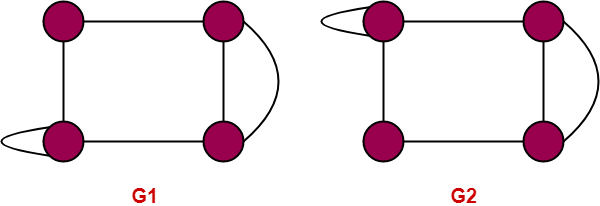## Solution-

### Condition-01:

• Number of vertices in graph G1 = 4
• Number of vertices in graph G2 = 4

Here,

• Both the graphs G1 and G2 have same number of vertices.
• So, Condition-01 satisfies.

### Condition-02:

• Number of edges in graph G1 = 5
• Number of edges in graph G2 = 6

Here,

• Both the graphs G1 and G2 have different number of edges.
• So, Condition-02 violates.

Since Condition-02 violates, so given graphs can not be isomorphic.

∴ G1 and G2 are not isomorphic graphs.

## Problem-02:

Which of the following graphs are isomorphic?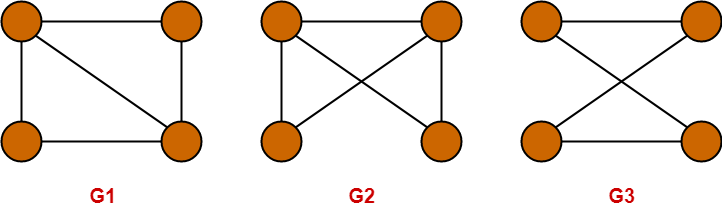## Solution-

### Condition-01:

• Number of vertices in graph G1 = 4
• Number of vertices in graph G2 = 4
• Number of vertices in graph G3 = 4

Here,

• All the graphs G1, G2 and G3 have same number of vertices.
• So, Condition-01 satisfies.

### Condition-02:

• Number of edges in graph G1 = 5
• Number of edges in graph G2 = 5
• Number of edges in graph G3 = 4

Here,

• The graphs G1 and G2 have same number of edges.
• So, Condition-02 satisfies for the graphs G1 and G2.
• However, the graphs (G1, G2) and G3 have different number of edges.
• So, Condition-02 violates for the graphs (G1, G2) and G3.

Since Condition-02 violates for the graphs (G1, G2) and G3, so they can not be isomorphic.

∴ G3 is neither isomorphic to G1 nor G2.

Since Condition-02 satisfies for the graphs G1 and G2, so they may be isomorphic.

∴ G1 may be isomorphic to G2.

Now, let us continue to check for the graphs G1 and G2.

### Condition-03:

• Degree Sequence of graph G1 = { 2 , 2 , 3 , 3 }
• Degree Sequence of graph G2 = { 2 , 2 , 3 , 3 }

Here,

• Both the graphs G1 and G2 have same degree sequence.
• So, Condition-03 satisfies.

### Condition-04:

• Both the graphs contain two cycles each of length 3 formed by the vertices having degrees { 2 , 3 , 3 }
• It means both the graphs G1 and G2 have same cycles in them.
• So, Condition-04 satisfies.

Thus,

• All the 4 necessary conditions are satisfied.
• So, graphs G1 and G2 may be isomorphic.

Now, let us check the sufficient condition.

### Checking Sufficient Condition-

We know that two graphs are surely isomorphic if and only if their complement graphs are isomorphic.

So, let us draw the complement graphs of G1 and G2.

The complement graphs of G1 and G2 are-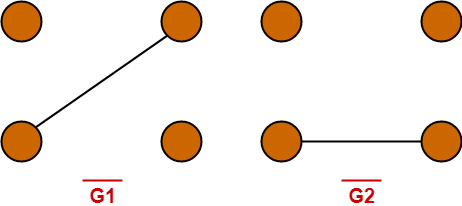Clearly, Complement graphs of G1 and G2 are isomorphic.

∴ Graphs G1 and G2 are isomorphic graphs.

## Problem-03:

Are the following two graphs isomorphic?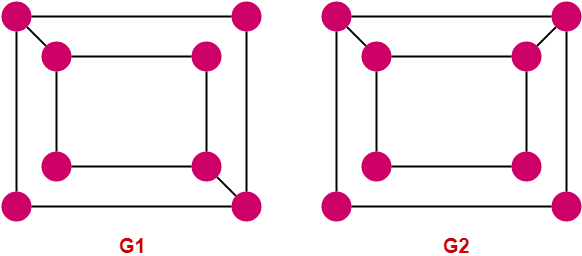## Solution-

### Condition-01:

• Number of vertices in graph G1 = 8
• Number of vertices in graph G2 = 8

Here,

• Both the graphs G1 and G2 have same number of vertices.
• So, Condition-01 satisfies.

### Condition-02:

• Number of edges in graph G1 = 10
• Number of edges in graph G2 = 10

Here,

• Both the graphs G1 and G2 have same number of edges.
• So, Condition-02 satisfies.

### Condition-03:

• Degree Sequence of graph G1 = { 2 , 2 , 2 , 2 , 3 , 3 , 3 , 3 }
• Degree Sequence of graph G2 = { 2 , 2 , 2 , 2 , 3 , 3 , 3 , 3 }

Here,

• Both the graphs G1 and G2 have same degree sequence.
• So, Condition-03 satisfies.

### Condition-04:

• In graph G1, degree-3 vertices form a cycle of length 4.
• In graph G2, degree-3 vertices do not form a 4-cycle as the vertices are not adjacent.

Here,

• Both the graphs G1 and G2 do not contain same cycles in them.
• So, Condition-04 violates.

Since Condition-04 violates, so given graphs can not be isomorphic.

∴ G1 and G2 are not isomorphic graphs.

To gain better understanding about Graph Isomorphism,

Watch this Video Lecture

Next Article-Complement of Graph

Get more notes and other study material of Graph Theory.

Watch video lectures by visiting our YouTube channel LearnVidFun.